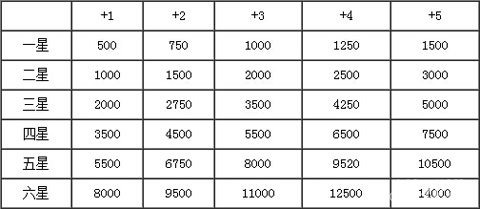# 七骑士不同星级强化所需金币详解攻略

2015-01-07 16:50:20来源：游戏狗整理编辑：吃猫的鱼2星+5，(1000+1500+2000+2500+3000)*2=10000*2=20000

3星+5，(2000+2750+3500+4250+5000)*2=17500*2=35000

4星+5，(3500+4500+5500+6500+7500)*2=27500*2=55000

5星+5，(5500+6750+8000+9520+10500)*2=40270*2=80540

6星+5，(8000+9500+11000+12500+14000)*2=55000*2=110000

3星+5，17500*4=70000

4星+5，27500*4=110000

5星+5，40270*4=161080

6星+5，55000*4=220000

4星+5，27500*10=275000

5星+5，40270*10=402700

6星+5，55000*10=550000